# Pandas DataFrame Plot area graph

Pandas plot

Pandas.DataFrame.plot to generate area using data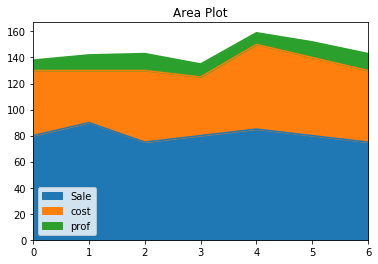``````import pandas as pd
my_dict={
'Sale':[80,90,75,80,85,80,75],
'cost':[50,40,55,45,65,60,55],
'prof':[8,12,13,10,9,12,13]
}
df =pd.DataFrame(data=my_dict)
df.plot.area(title='Area Plot')``````

## area chart with options

There are several options we can add to above area diagram.

## title :

title='Area Plot' String used as Title of the graph.

## figsize :Size of the graph , it is a tuple saying width and height in inches, figsize=(6,3). Here width is 6 inches and height is 3 inches.
``df.plot.area(figsize=(6,3))``

## fontsize

fontsize=20 , we can set the font size used labels in x and y axis.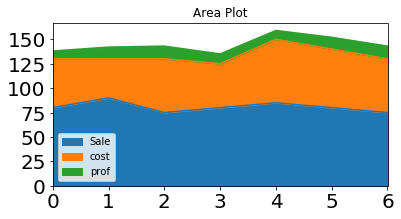``df.plot.area(fontsize=20)``

## color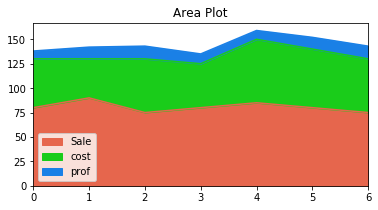We can use the option colors to give different colors to line . We can use one tuple to define the colours.

``````my_color=[(.9,.4,.3),(.1,.8,.1),(.1,.5,.9)]
df.plot.area(title='Area Plot',color=my_color)``````

## style

This is a list or dict. We can specify how the style of the line.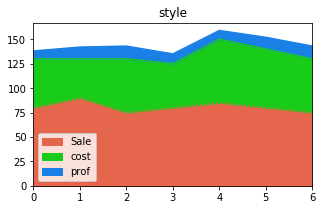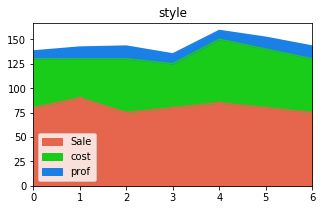``````#my_style=[':']
my_style=['.-']
df.plot.area(title='style',style=my_style)``````

## grid

We will show grid ( grid=True ) or not ( grid=False)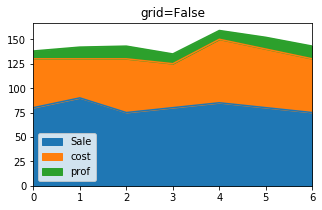``df.plot.area(title='grid=True',grid=True)``

## logxWe can specify log scaling or symlog scaling for x ( logx=True )

``df.plot.area(logx=True)``

## secondary_y

Whether to plot on secondary Y Axis ( secondary_y=True ) or not ( secondary_y=False )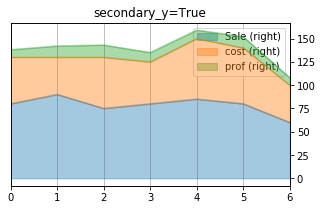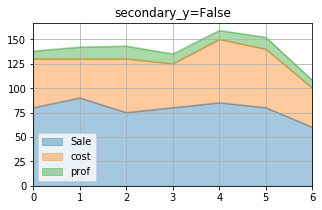``df.plot.area(secondary_y=True)``

## mark_right

Check the image above when secondary_y=True. There is a automatic marking in column lebels saying (right). We can manage this to show ( mark_right=True) or not ( mark_right=False)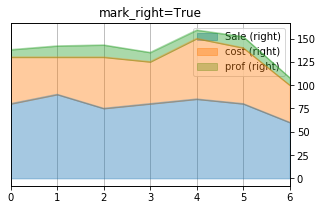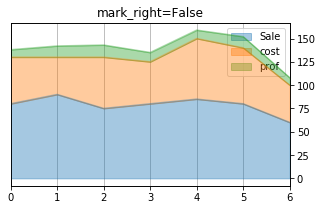``df.plot.area(title='mark_right=False',secondary_y=True,mark_right=True,alpha=.4)``

## rot

Rotation of ticks ( check the label at x axis )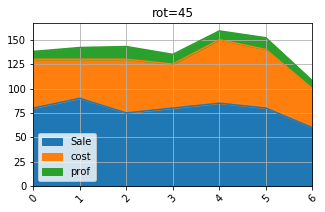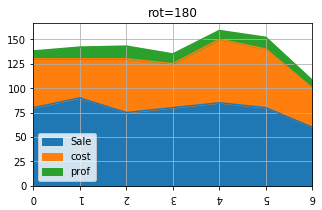``df.plot.area(title='rot=180',rot=180)``

Subscribe to our YouTube Channel here

## Subscribe

* indicates required
Subscribe to plus2netplus2net.com